## 1.线性表的定义和基本操作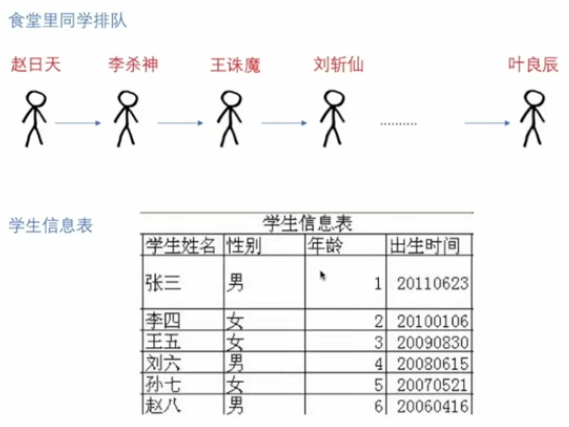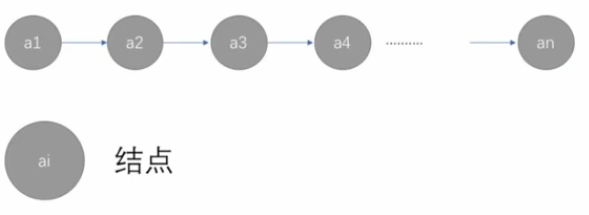## 2.线性表的顺序存储结构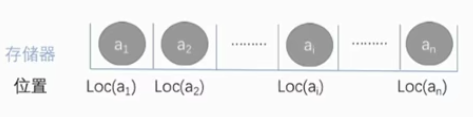Loc(a2)=Loc(a1)+d d是元素占据的存储单元数

Loc(ai)=Loca(a1)+(i-1)*d

### 2.1如何建立线性表的顺序存储结构

• 1.存储空间的起始位置
• 2.顺序表最大存储容量
• 3.顺序表当前的长度
#define MaxSize 50  //定义线性表的最大长度
typedef int Elemtype   //假定表中元素类型是int
typedef struct{
ElemType data [MaxSize];   //顺序表的元素(数组)
int length;         //顺序表的当前长度
}SqList;                   //顺序表的类型定义


typedef int Elemtype;
typedef struct{
ElemType  *data;  //指示动态分配数组的指针
int MaxSize,length; //数组的最大容量和当前个数
}SeqList;
//动态分配语句(c语言)
#define initSize 100
SeqList L;
L.data(ElemType*)malloc(sizeof(ElemType)*initSize);


## 3.总结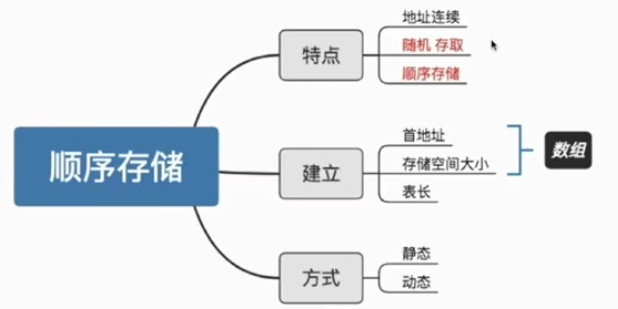## 4.顺序表的操作

### 4.1插入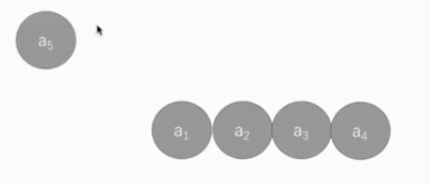a5的位置在a1和a2之间。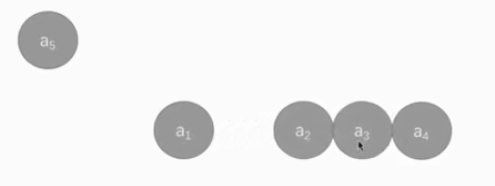bool是布尔类型它只有true和 false两种值,分别表示真和假

• 1.判断的值是否正确
• 2.判断表长是否超过数组长度
• 3.从后向前到第个位置,分别将这些元素都向后移动一位
• 4.将元素插入位置 i ，修改表长
bool ListInsert(SqList &L,int i,ElemType e){
if(i<1||i>L.length+1){ //判断i的范围是否有效
return false;
}
if(L.length>=MaxSize){  //当前存储空间已经满，不能插入
return false;
}
for(int j=L.length;j>=i;j--){ //将第i个元素之后的元素后移
//初值为下标为L.length是因为要将最后一位向后移动，L.length代表移0动后的下标L.length-1代表当前数组中最后一个元素。
//j>=i,是因为i代表位置i-1才代表最终要移动到的位置的下标，将第i的元素的值变为第i-1个元素的值，就是后移。
L.data[j]=L.data[j-1]
}
L.data[i-1] = e; //在位置 i 插入e
L.length++;  //数组长度加1
return true;
}



好情况：在表尾插入(即i=n+1),元素后移语句将不执行,时间复杂度为O(1)。
坏情况：在表头插入(即i=1),元素后移语句将执行n次,时间复杂度为O(n)
平均情况：假设 p i( pi=1/(n+1) [1/(n+1)是因为总共有n+1一个位置可以被插入]) 是在第 i 个位置上插入一个结点的概率,则在长度为n的线性表中插入一个结点时所需移动结点的平均次数为: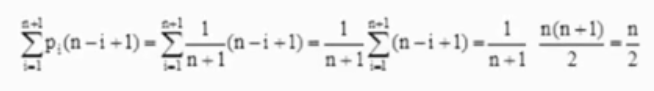### 4.1删除• 1.判断的值是否正确
• 2.取删除的元素
• 3.将被删元素后面的所有元素都依次向前移动一位
• 4.修改表长
bool ListDelete(SqList &L,int i,Elemtype &e){
if(i<1||i>L.length){ //判断 i 是否有效
return false;
}
e=L.data[i-1]; //将被删除的元素复制给e
for(int j=i;j<L.length;j++){
//将第i个位置之后的元素前移
//注意：i 代表位置，不是下标
L.data[j-1] = L.data[j];
}
L.length
return true;
}


好情况：删除表尾元素(即i=n),无须移动元素,时间复杂度为O(1)
坏情况：删除表头元素(即i=1)需要移动除第一个元素外的所有元素,时间复杂度为O(n)
平均情况：假设pi(pi=1/n [1/n是因为总共有n个位置可以被删除])是删除第 i 个位置上结点的概率，则在长度为n的线性表中删除一个结点时所需移动结点的平均次数为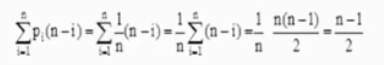## 5.总结

顺序存储优点

• 存储密度大:不需要为表中元素之间的逻辑关系增加额外存储空间。
• 随机存取:可以快速存取表中任一位置的元素

顺序存储缺点

• 插入和删除操作需要移动大量元素
• 对存储空间要求高,会产生存储空间的“碎片”

#### 欢迎关注公众号 理木客 更多精彩等你发现